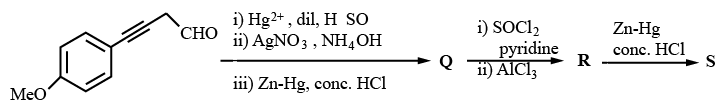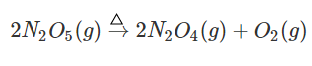Instructions

For the following questions answer them individually

Question 21

# Consider the following reactions (unbalanced)Zn + hot conc.$$H_2SO_4 \rightarrow G + R + X$$Zn + conc.$$NaOH \rightarrow T + Q$$G + $$H_2S + NH_4OH \rightarrow Z$$ (a precipitate) + X + YChoose the correct option(s)Question 22

# The ground state energy of hydrogen atom is —13.6 eV. Consider an electronic state $$\psi$$ of $$He^+$$ whose energy, azimuthal quantum number and magnetic quantum number are —3.4 eV, 2 and 0, respectively. Which of the following statement(s) is(are) true for the state $$\psi$$?Question 23

# Which of the following reactions produce(s) propane as a major product?Question 24

# Choose the correct option(s) that give(s) an aromatic compound as the major productQuestion 25

# Choose the correct option(s) for the following reaction sequenceConsider Q, R and S as major productsQuestion 26

# Choose the correct option(s) from the followingQuestion 27

# The amount of water produced (in g) in the oxidation of 1 mole of rhombic sulphur by conc. $$HNO_3$$ to a compound with the highest oxidation state of sulphur is ___________ .(Given data: Molar mass of water = $$18 g mol^{-1}$$)

Backspace
789
456
123
0.-
Clear AllQuestion 28

# Total number of c is N-Mn-Cl bond angles (that is, Mn-N and Mn-Cl bonds in c is positions) present in a molecule of c is-$$[Mn(en)_2 Cl_2]$$ complex is (en = $$NH_2CH_2CH_2NH_2)$$

Backspace
789
456
123
0.-
Clear AllQuestion 29

# The decomposition reactionis started in a closed cylinder under isothermal isochoric condition at an initial pressure of 1 atm. After $$Y \times 10^3 s$$, the pressure inside the cylinder is found to be 1.45 atm. If the rate constant of the reaction is $$5 \times 10^{-4} s^{-1}$$, assuming ideal gas behavior, the value of Y is __________ .

Backspace
789
456
123
0.-
Clear AllQuestion 30

# The mole fraction of urea in an aqueous urea solution containing 900 g of water is 0.05. If the density of the solution is $$1.2 g cm^{-3}, the molarity of urea solution is(Given data: Molar masses of urea and water are$$60 g mol^{-1}$$and$$18 g mol^{-1}, respectively)

Backspace
789
456
123
0.-
Clear AllOR